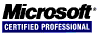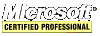## strcpy in x86 Assembly

This procedure, `strcpy_asm`, copies a C-string from the source to the destination and returns a pointer to the end of the destination so that another string can be concatenated. For Unicode strings see `wcscpy_asm`.

The default behavior of `strcpy` is to return the destination buffer but it is much more useful to return the end of the text in the destination buffer.

``````TITLE 'extern "C" char * strcpy_asm(char *destination, const char *source);'

.686

.model FLAT

PUBLIC	_strcpy_asm

_STRCPY	SEGMENT
_strcpy_asm PROC NEAR

mov  edx, DWORD PTR [esp+8] ; source
mov  cl, BYTE PTR [edx]
test cl, cl
mov  eax, DWORD PTR [esp+4] ; destination
je   SHORT label2

label1:

mov  BYTE PTR [eax], cl
mov  cl, BYTE PTR [edx+1]
inc  eax
inc  edx
test cl, cl
jne  SHORT label1

label2:

mov  BYTE PTR [eax], 0

ret  0

_strcpy_asm ENDP
_STRCPY	ENDS
END
``````

This example C++ code uses this function.

``````#include <stdlib.h>

extern "C" char * strcpy_asm(char *destination, const char *source);

char str, *end;
int length;

end = strcpy_asm(str, "How ");
end = strcpy_asm(end, "now ");
end = strcpy_asm(end, "brown ");
end = strcpy_asm(end, "cow!");

length = end - str;

// or simply

length = strcpy_asm(strcpy_asm(strcpy_asm(strcpy_asm(str, "How "), "now "), "brown "), "cow!") - str;

// str == "How now brown cow!"
// length == 18
``````